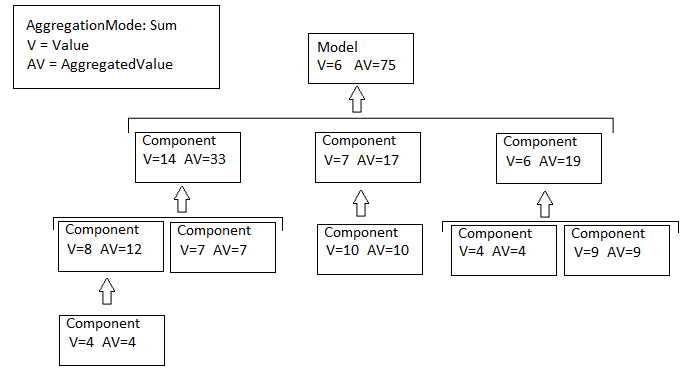Documentation

Model Metric Data Aggregation

You can better understand the size, complexity, and readability of a model and its components by analyzing aggregated model metric data. Aggregated metric data is available in the AggregatedValue and AggregatedMeasures properties of an slmetric.metric.Result object. The AggregatedValue property aggregates the metric scalar values. The AggregatedMeasures property aggregates the metric measures (that is, the detailed information about the metric values).

How Model Metric Aggregation Works

The implementation of a model metric defines how a metric aggregates data across a component hierarchy. For MathWorks model metrics, the slmetric.metric.Metric class defines model metric aggregation. This class includes these two aggregation properties:

• AggregationMode, which has these options:

• Sum: Returns the sum of the Value property and the Value properties of its children components across the component hierarchy. Returns the sum of the Meaures property and the Measures properties of its children components across the component hierarchy.

• Max: Returns the maximum of the Value property and the Value properties of its children components across the component hierarchy. Returns the maximum of the Measures property and the Measures properties of its children components across the component hierarchy.

• None: No aggregation of metric values.

• AggregateComponentDetails is a Boolean value, which has these options:

• true: For metrics that return fine-granular results (that is, more than one result per component), the software aggregates these results to the component level by taking the sum of the values and measures properties. Returns a result that spans the complete component.

• false: Returns the component results. The software does not aggregate the fine-granular results.

The MathWorks model metrics that return fine-granular results are:

You can find descriptions of MathWorks model metrics and their aggregation property settings in Model Metrics. For custom metrics, as part of the algorithm method, you can define how the metric aggregates data. For more information, see Create a Custom Model Metric for Nonvirtual Block Count.

This diagram shows how the software aggregates metric data across the components of a model hierarchy. The parent model is at the top of the hierarchy. The components can be the following:

• Model

• Subsystem block

• Chart

• MATLAB function block

• Protected modelAccess Aggregated Metric Data

This example shows how to collect metric data programmatically in the metric engine, and then access aggregated metric data.

1. Load the sldemo_applyVarStruct model.

model = 'sldemo_applyVarStruct';
open(model);
2. Create an slmetric.Engine object and set the analysis root.

metric_engine = slmetric.Engine();
setAnalysisRoot(metric_engine,'Root',model,'RootType','Model');

3. Collect data for the Input output model metric.

execute(metric_engine,'mathworks.metrics.IOCount');
4. Get the model metric data that returns an array of slmetric.metric.ResultCollection objects, res_col. Specify the input argument for AggregationDepth.

res_col = getMetrics(metric_engine,'mathworks.metrics.IOCount',...
'AggregationDepth','All');

The AggregationDepth input argument has two options: All and None. If you do not want the getMetrics method to aggregate measures and values, specify None.

5. Display the results.

metricData ={'MetricID','ComponentPath','Value',...
'AggregatedValue','Measures','AggregatedMeasures'};
cnt = 1;
for n=1:length(res_col)
if res_col(n).Status == 0
results = res_col(n).Results;

for m=1:length(results)
disp(['MetricID: ',results(m).MetricID]);
disp(['  ComponentPath: ',results(m).ComponentPath]);
disp(['  Value: ',num2str(results(m).Value)]);
disp(['  Aggregated Value: ',num2str(results(m).AggregatedValue)]);
disp(['  Measures: ',num2str(results(m).Measures)]);
disp(['  Aggregated Measures: ',...
num2str(results(m).AggregatedMeasures)]);
metricData{cnt+1,1} = results(m).MetricID;
metricData{cnt+1,2} = results(m).ComponentPath;
metricData{cnt+1,3} = results(m).Value;
tdmetricData{cnt+1,4} = results(m).Measures;
metricData{cnt+1,5} = results(m).AggregatedMeasures;
cnt = cnt + 1;
end
else
end
disp(' ');
end

Here are the results:

MetricID: mathworks.metrics.IOCount
ComponentPath: sldemo_applyVarStruct
Value: 3
Aggregated Value: 5
Measures: 1  2  0  0
Aggregated Measures: 3  2  0  0
MetricID: mathworks.metrics.IOCount
ComponentPath: sldemo_applyVarStruct/Controller
Value: 4
Aggregated Value: 4
Measures: 3  1  0  0
Aggregated Measures: 3  1  0  0
MetricID: mathworks.metrics.IOCount
ComponentPath: sldemo_applyVarStruct/Aircraft
Dynamics
Model
Value: 5
Aggregated Value: 5
Measures: 3  2  0  0
Aggregated Measures: 3  2  0  0
MetricID: mathworks.metrics.IOCount
ComponentPath: sldemo_applyVarStruct/Dryden Wind
Gust Models
Value: 2
Aggregated Value: 2
Measures: 0  2  0  0
Aggregated Measures: 0  2  0  0
MetricID: mathworks.metrics.IOCount
ComponentPath: sldemo_applyVarStruct/Nz pilot
calculation
Value: 3
Aggregated Value: 3
Measures: 2  1  0  0
Aggregated Measures: 2  1  0  0
MetricID: mathworks.metrics.IOCount
Value: 0
Aggregated Value: 0
Measures: 0  0  0  0
Aggregated Measures: 0  0  0  0

For the Input output metric, the AggregationMode is Max. For each component, the AggregatedValue and AggregatedMeasures properties are the maximum number of inputs and outputs of itself and its children components. For example, for sldemo_applyVarStruct, the AggregatedValue property is 5, which is the sldemo_applyVarStruct/Aircraft Dynamics Model component value.